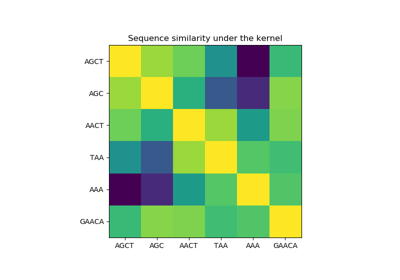# sklearn.gaussian_process.kernels.Kernel¶

class sklearn.gaussian_process.kernels.Kernel[source]

Base class for all kernels.

New in version 0.18.

Attributes
bounds

Returns the log-transformed bounds on the theta.

hyperparameters

Returns a list of all hyperparameter specifications.

n_dims

Returns the number of non-fixed hyperparameters of the kernel.

requires_vector_input

Returns whether the kernel is defined on fixed-length feature vectors or generic objects.

theta

Returns the (flattened, log-transformed) non-fixed hyperparameters.

Methods

 __call__(self, X[, Y, eval_gradient]) Evaluate the kernel. clone_with_theta(self, theta) Returns a clone of self with given hyperparameters theta. diag(self, X) Returns the diagonal of the kernel k(X, X). get_params(self[, deep]) Get parameters of this kernel. is_stationary(self) Returns whether the kernel is stationary. set_params(self, \*\*params) Set the parameters of this kernel.
__init__(self, /, *args, **kwargs)

Initialize self. See help(type(self)) for accurate signature.

abstract __call__(self, X, Y=None, eval_gradient=False)[source]

Evaluate the kernel.

property bounds

Returns the log-transformed bounds on the theta.

Returns
boundsarray, shape (n_dims, 2)

The log-transformed bounds on the kernel’s hyperparameters theta

clone_with_theta(self, theta)[source]

Returns a clone of self with given hyperparameters theta.

Parameters
thetaarray, shape (n_dims,)

The hyperparameters

abstract diag(self, X)[source]

Returns the diagonal of the kernel k(X, X).

The result of this method is identical to np.diag(self(X)); however, it can be evaluated more efficiently since only the diagonal is evaluated.

Parameters
Xsequence of length n_samples

Left argument of the returned kernel k(X, Y)

Returns
K_diagarray, shape (n_samples_X,)

Diagonal of kernel k(X, X)

get_params(self, deep=True)[source]

Get parameters of this kernel.

Parameters
deepboolean, optional

If True, will return the parameters for this estimator and contained subobjects that are estimators.

Returns
paramsmapping of string to any

Parameter names mapped to their values.

property hyperparameters

Returns a list of all hyperparameter specifications.

abstract is_stationary(self)[source]

Returns whether the kernel is stationary.

property n_dims

Returns the number of non-fixed hyperparameters of the kernel.

property requires_vector_input

Returns whether the kernel is defined on fixed-length feature vectors or generic objects. Defaults to True for backward compatibility.

set_params(self, **params)[source]

Set the parameters of this kernel.

The method works on simple kernels as well as on nested kernels. The latter have parameters of the form <component>__<parameter> so that it’s possible to update each component of a nested object.

Returns
self
property theta

Returns the (flattened, log-transformed) non-fixed hyperparameters.

Note that theta are typically the log-transformed values of the kernel’s hyperparameters as this representation of the search space is more amenable for hyperparameter search, as hyperparameters like length-scales naturally live on a log-scale.

Returns
thetaarray, shape (n_dims,)

The non-fixed, log-transformed hyperparameters of the kernel

## Examples using sklearn.gaussian_process.kernels.Kernel¶